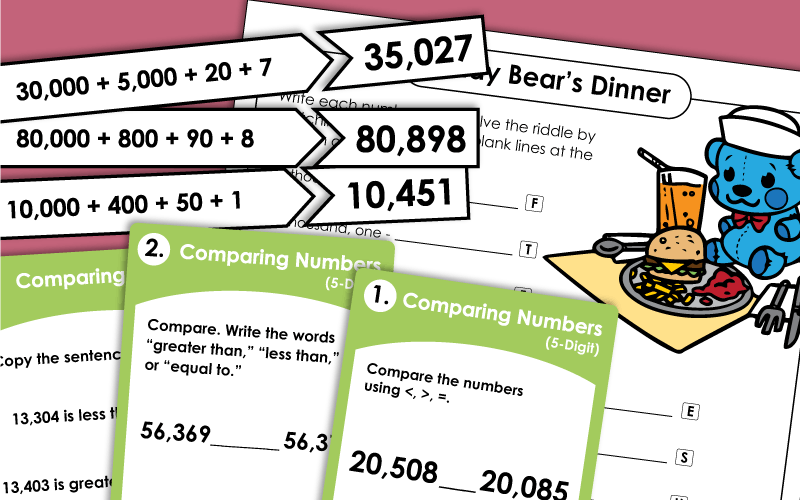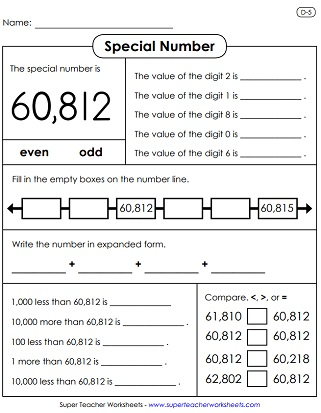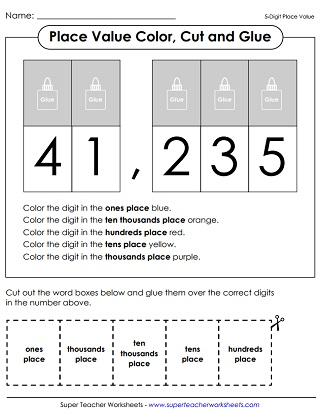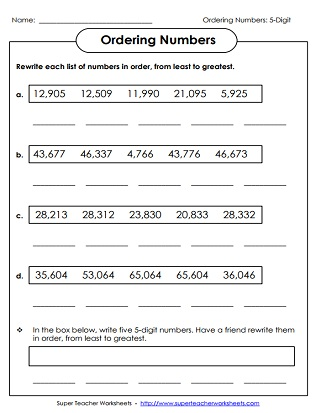# Place Value: 5 Digits (Ten Thousands)

Worksheets for teaching place value of 5-digit numbers. Use these resources to learn about writing numbers in expanded form, ordering numbers from least to greatest, reading large numbers, placing commas in numbers, and determining the values of underlined digits.## Place Value

Carefully cut the word cards (ones, tens, hundreds, thousands, and ten thousands). Look at the large 5-digit number on the page and glue the word cards over the correct digit.
Fill in the place-value chart for the 5-digit numbers given. Then write them out in expanded and word form.
This worksheet has 6 more 5-digit numbers for students to write in expanded and word form and complete the place-value tables.
Print these puzzle pieces to match numbers to their expanded form. Numbers up to the ten thousands place.
What did the teddy bear say after she had finished her supper? To find out, write the 5-digit numbers in standard form. Then match the numbers with the letters in the solution.
Students are given sheets of paper with large digits printed on them.  The teacher says a number. (example: fifteen thousands, sixty) Students arrange the digits to make the number.
In this game, a teacher attaches a place value task card to each desk in the classroom. Students write the answer to the question on each card as they "scoot" around the room.
Cut out the pieces and organize them into groups of equal numbers. Also, classify each as standard form, word name, or expanded form.
Why was six scared of seven? To find out, write the value of the underlined digits.
Use the clues to figure out each mystery number.
Write the ancient Egyptian numbers using the chart. Surprisingly, Ancient Egyptians' place value system worked a lot like our own number system.
Read and write large numbers with this five-digit place value table.
This page can be handed out to students or hung in the classroom to use as a reference for five-digit place value. It shows an example for how to write a number in expanded form and notation, as well as standard and written form.

## Special Numbers

Write the special number is expanded form. Then tell whether this number is odd or even. Write the numbers that come before and after on the number line. State the values of the digits 2, 4, 6, and 9.
Answer a series of place-value questions that have to do with the number thirty thousand, eight hundred forty.
Answer the place value questions for the number nineteen thousand, five hundred.
Write the values of the digits, and tell if the number is odd or even. Compare other numbers to this number. Write in expanded form.
What are the values of the digits 2, 1, 8, and 6? Compare the special number to other numbers, using <, >, and =. Complete the number line.

## Ordering Numbers

Students cut the five-digit number cards and lay them out on their desk in order from least to greatest.
Arrange the each of the numbers in the order, from the least to the greatest.

## Comparing Numbers

Use mathematical symbols <, >, and = to compare the values of the given numbers. This worksheet also includes word problems.
This file has 30 task cards for comparing 5-digit numbers. These can be used for classroom games, with your document camera, for small-group instruction, or for learning centers.

Teachers score students as they read aloud these 5-digit numbers on the included score sheets.
How many of these 5-digit numbers can your students correctly say?
Spin the number wheel and read each number aloud to a teacher, classmate, or parent.
6-Digit Place Value

Review 6-digit place value with these games, worksheets, and lessons.

## Pictures of Our WorksheetsMy Account
Site Information